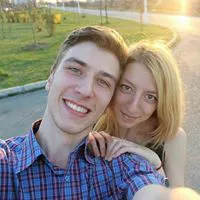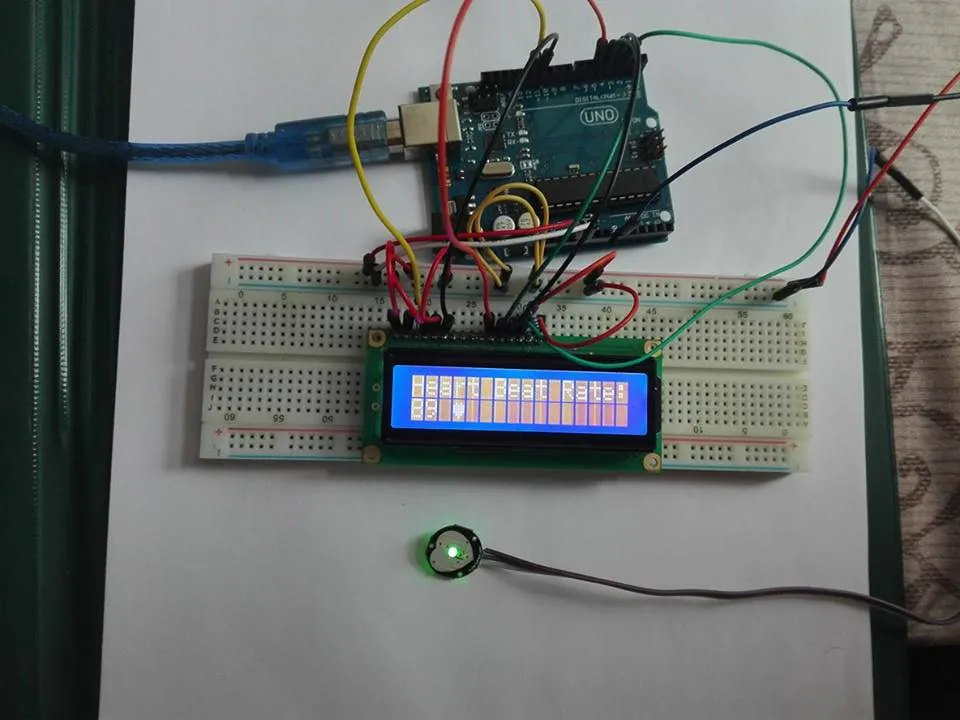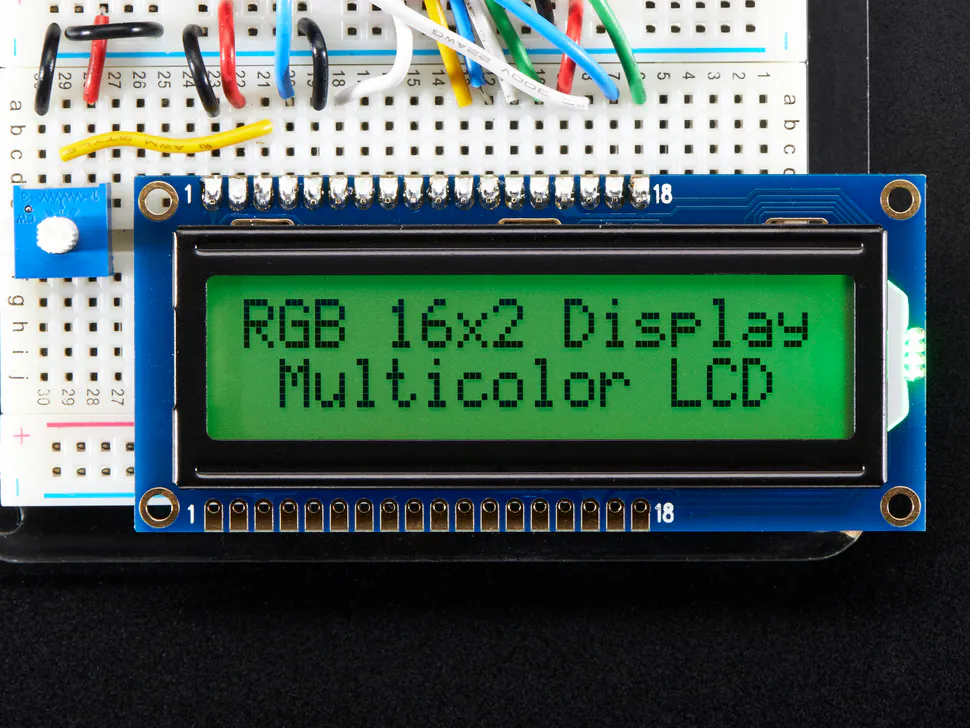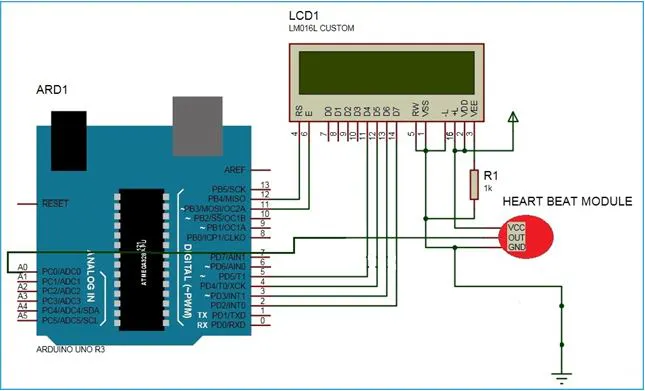Published

# Cardiac Monitoring System - EKG

I have used a heartbeat sensor module which senses the heartbeat upon putting a finger on the sensor.

IntermediateShowcase (no instructions)4 hours27,520## Things used in this project

### Hardware componentsArduino UNO
×1Adafruit RGB Backlight LCD - 16x2
×1
 SparkFun heart rate sensor
×1Resistor 10k ohm
×1Jumper wires (generic)
×1×1

### Software apps and online services

 Processing

## Schematics

### Circuit Diagram## Code

### Arduino-code

Arduino
This is the code from Arduino and you have to uploaded on your Arduino device.
```#include<LiquidCrystal.h>

LiquidCrystal lcd(12, 11, 5, 4, 3, 2);

byte heart =
{
0b00000,
0b01010,
0b11111,
0b11111,
0b11111,
0b01110,
0b00100,
0b00000
};

//  VARIABLES
int pulsePin = 0;                 // Pulse Sensor purple wire connected to analog pin 0
int blinkPin = 13;                // pin to blink led at each beat

// these variables are volatile because they are used during the interrupt service routine!
volatile int BPM;                   // used to hold the pulse rate
volatile int Signal;                // holds the incoming raw data
volatile int IBI = 600;             // holds the time between beats, the Inter-Beat Interval
volatile boolean Pulse = false;     // true when pulse wave is high, false when it's low
volatile boolean QS = false;        // becomes true when Arduoino finds a beat.

void setup(){
Serial.begin(115200);             //The speed of communication
interruptSetup();

lcd.createChar(1, heart);
lcd.begin(16,2);
lcd.print("Heart Beat ");
lcd.write(1);
lcd.setCursor(0,1);
lcd.print("Monitering");
pinMode(pulsePin, INPUT);
delay(1000);
}

void loop(){
sendDataToProcessing('S', Signal);     // send Processing the raw Pulse Sensor data
if (QS == true){                       // Quantified Self flag is true when arduino finds a heartbeat
sendDataToProcessing('B',BPM);   // send heart rate with a 'B' prefix
QS = false;                      // reset the Quantified Self flag for next time
}
delay(20);
}

void sendDataToProcessing(char symbol, int data ){
Serial.print(symbol);                // symbol prefix tells Processing what type of data is coming
Serial.println(data);                // the data to send culminating in a carriage return
}

volatile int rate;                    // used to hold last ten IBI values
volatile unsigned long sampleCounter = 0;          // used to determine pulse timing
volatile unsigned long lastBeatTime = 0;           // used to find the inter beat interval
volatile int P =512;                      // used to find peak in pulse wave
volatile int T = 512;                     // used to find trough in pulse wave
volatile int thresh = 512;                // used to find instant moment of heart beat
volatile int amp = 100;                   // used to hold amplitude of pulse waveform
volatile boolean firstBeat = true;        // used to seed rate array so we startup with reasonable BPM
volatile boolean secondBeat = true;       // used to seed rate array so we startup with reasonable BPM

void interruptSetup(){
// Initializes Timer2 to throw an interrupt every 2mS.
TCCR2A = 0x02;     // DISABLE PWM ON DIGITAL PINS 3 AND 11, AND GO INTO CTC MODE
TCCR2B = 0x06;     // DON'T FORCE COMPARE, 256 PRESCALER
OCR2A = 0X7C;      // SET THE TOP OF THE COUNT TO 124 FOR 500Hz SAMPLE RATE
TIMSK2 = 0x02;     // ENABLE INTERRUPT ON MATCH BETWEEN TIMER2 AND OCR2A
sei();             // MAKE SURE GLOBAL INTERRUPTS ARE ENABLED
}

// THIS IS THE TIMER 2 INTERRUPT SERVICE ROUTINE.
// Timer 2 makes sure that we take a reading every 2 miliseconds
ISR(TIMER2_COMPA_vect){                         // triggered when Timer2 counts to 124
cli();                                      // disable interrupts while we do this
sampleCounter += 2;                         // keep track of the time in mS with this variable
int N = sampleCounter - lastBeatTime;       // monitor the time since the last beat to avoid noise

//  find the peak and trough of the pulse wave
if(Signal < thresh && N > (IBI/5)*3){       // avoid dichrotic noise by waiting 3/5 of last IBI
if (Signal < T){                        // T is the trough
T = Signal;                         // keep track of lowest point in pulse wave
}
}

if(Signal > thresh && Signal > P){          // thresh condition helps avoid noise
P = Signal;                             // P is the peak
}                                        // keep track of highest point in pulse wave

//  Cautare semnal de puls
// signal surges up in value every time there is a pulse
if (N > 250){                                   // avoid high frequency noise
if ( (Signal > thresh) && (Pulse == false) && (N > (IBI/5)*3) ){
Pulse = true;                               // set the Pulse flag when we think there is a pulse
digitalWrite(blinkPin,HIGH);                // turn on pin 13 LED
IBI = sampleCounter - lastBeatTime;         // measure time between beats in mS
lastBeatTime = sampleCounter;               // keep track of time for next pulse

if(firstBeat){                         // if it's the first time we found a beat, if firstBeat == TRUE
firstBeat = false;                 // clear firstBeat flag
return;                            // IBI value is unreliable so discard it
}
if(secondBeat){                        // if this is the second beat, if secondBeat == TRUE
secondBeat = false;                 // clear secondBeat flag
for(int i=0; i<=9; i++){         // seed the running total to get a realisitic BPM at startup
rate[i] = IBI;
}
}

// keep a running total of the last 10 IBI values
word runningTotal = 0;                   // clear the runningTotal variable

for(int i=0; i<=8; i++){                // shift data in the rate array
rate[i] = rate[i+1];              // and drop the oldest IBI value
runningTotal += rate[i];          // add up the 9 oldest IBI values
}

rate = IBI;                          // add the latest IBI to the rate array
runningTotal += rate;                // add the latest IBI to runningTotal
runningTotal /= 10;                     // average the last 10 IBI values
BPM = 60000/runningTotal;               // how many beats can fit into a minute? that's BPM!
lcd.clear();
lcd.print("Heart Beat Rate:");
lcd.setCursor(0,1);
lcd.print(BPM);
lcd.print(" ");
lcd.write(1);
QS = true;                              // set Quantified Self flag
// QS FLAG IS NOT CLEARED INSIDE THIS ISR
}
}

if (Signal < thresh && Pulse == true){     // when the values are going down, the beat is over
digitalWrite(blinkPin,LOW);            // turn off pin 13 LED
Pulse = false;                         // reset the Pulse flag so we can do it again
amp = P - T;                           // get amplitude of the pulse wave
thresh = amp/2 + T;                    // set thresh at 50% of the amplitude
P = thresh;                            // reset these for next time
T = thresh;
}

if (N > 2500){                             // if 2.5 seconds go by without a beat
thresh = 512;                          // set thresh default
P = 512;                               // set P default
T = 512;                               // set T default
lastBeatTime = sampleCounter;          // bring the lastBeatTime up to date
firstBeat = true;                      // set these to avoid noise
secondBeat = true;                     // when we get the heartbeat back
}

sei();                                     // enable interrupts when youre done!
}
```